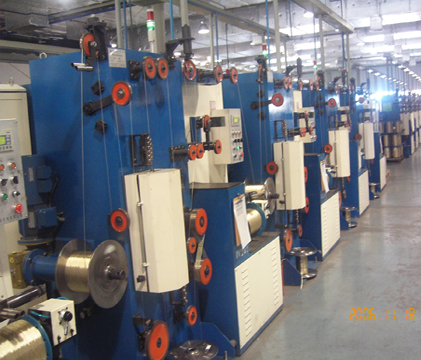## 艾默生TD3300变频器与三菱PLC可编程控制器在高速微拉丝机应用调试报告1 拉丝机双变频控制系统

1.1系统配置：主拉为11kW/EV2000，收线采用4kW/TD3300。电机都为2极电机。最高速2880r/min，最高频率50hz；配有电磁刹车。
1.2工艺要求：
（1）最高收线速度2000m/min；
（2）收卷卷径223-300mm。
（3）进线0.9mm，出线0.15mm，铜丝
1.3控制原理：

2.调试说明：

2.1 启动开始阶段，收线摆干往上翘，直到把线拉断了，分析是
A、由于收线电机的速度突然上升太快的原因。由于收线电机开机时速度的偏差比较大，在进行调解的时候调解的量过大，找到机械上因为电磁刹车片放开的时候还有一定的摩擦力，导致收卷电机启动上就慢一步，通过调整刹车片，解决这个方面的问题
B、还有就是同步频率没有调整好，通过调整最大线速度，使变频器运算的线速度和实际的线速度更接近。
2.2启动后拖着摆杆感觉又往下压的力量。

2.3 拉丝电机升速到设定值的一刻，摆杆有个比较大的波动，有时大的波动可能短线或者判断为断线。

2.4停车过程中，收卷电机总是比拉丝电机晚停止，造成线被拉断。

2.5启动停车时，拉丝电机的加减速时间不可控

2.6启动后发现摆杆上去后又降下，手托住后有上升，让后还是会降下。

3，调试总结
3.1、影响调试进度的原因有很多，包括很多的机械的和外围电器的原因，如果仅从变频器去调节，可能永远也达不到目的，所以要多注意从现象先去排除外围的影响。在拉丝机调试中，发现有一些参数是比较重要的：最大线速度、最小线速度、拉丝电机的加减速时间、卷径计算、卷径滤波、pid参考量为卷径。
3.2、调试最终现象结果：

Ev2000主要修改参数
F0.00=3 F7.00=9 F7.10=2 F7.13=0.5 F7.15=0
F7.30=96 FH.00=2
F0.09=2.5转矩提升

TD3300主要修改参数
F0.02=1 F0.03=0 F0.05=1 F0.07=50 F0.08=50 F0.09=0.5 F0.10=0.5 F0.11=0.5
F1.00=0 F1.01=4.0
F7.00=0 F7.02=2 F7.03=10 F7.04=3 F7.05=1.0 F7.06=12 F7.07=2.5 F7.08=0.95 F7.09=0 F7.10=0 F7.11=0.1 F7.12=50 F7.13=100
F8.00=0 F8.01=0 F8.02=50 F8.03=100 F8.04=4 F8.06=0 F8.07=0 F8.08=1 F8.09=300 F8.10=223 F8.11=0 F8.12=223 F8.17=0
FA.10=7
FC.00=2 FC.01=0 FC.02=0 FC.03=1940 FC.04=500 FC.05=9.99